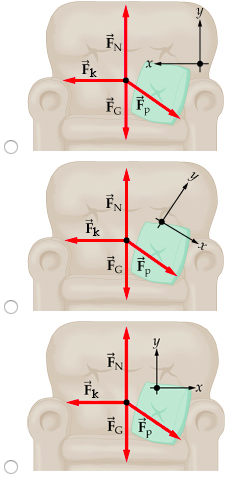# Problem: Which set of coordinate axes is the most convenient to use in this problem?

###### FREE Expert Solution

Usually, the coordinates with a positive x-axis and positive y-axis form the basis of solving vectors.

Such a coordinate system is described by the x-component towards the right being taken as positive and the x-component towards the left being negative.

84% (468 ratings)###### Problem Details

Which set of coordinate axes is the most convenient to use in this problem?